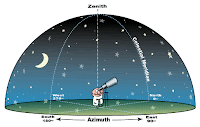## Friday, April 17, 2009

### Celestial Sphere

Celestial Sphere

Lecturer – J D Prasanna Deshapriya
Event – Observation Camp of Asian International School on March 30, 2009

Have you ever wondered how far the starts really are away from us? Well, what are the closest and most distant stars? If you could provide a better solution for above questions, you are not that far from the correct answer.

If you observe the night skies, it seems that all the stars are same distance away from us. Therefore to make it easy to study, we assume that all the stars lie in a surface of a sphere, whose center is concentric with that of Earth. This sphere is known as “Celestial Sphere”

It is important to understand that each star occupies a unique location of its own, as same as a country does, in the surface of the Earth. The celestial sphere is divided in to 88 parts, each of which contains a constellation.

To identify a location of celestial sphere, we use co-ordination system as same as latitudes and longitudes are used to determine the geographical locations.

Let’s get known more about the geographical co-ordination system. The Earth is divided into two equal parts by the equator. The upper part is called Northern Hemisphere where as the portion, below the equator is called Southern Hemisphere. The lines that are drawn in parallel to the equator are called latitudes and the lines that are perpendicular to the equator are called longitudes. Using this co-ordination system we could explain any single point on the surface of the Earth, since each location has its own attributes of above-mentioned values (latitude & longitude).

Co-ordination Systems of Celestial Sphere

Now, let’s derive a method to explain a location of celestial sphere. In fact thereare two systems in use. These systems help us determine the locations of the stars on the celestial surface.

1. Equatorial Co-ordination system (RA-Dec)

If you could project the attributes of previously discussed terrestrial co-ordination system to the celestial sphere you would get a formal system of co-ordinates for the celestial sphere as well. The celestial equivalent of the equator will be Celestial Equator, where as corresponding components of the latitudes and longitudes will be Declination and Right Ascension (RA-Dec), respectively. Therefore the location of a star will be given in RA and Dec in this system which is known as Equatorial Co-ordination system.

The measurement of declination will be dealt with angles. The values of the declination will be measured as positive (+) if the object/ star is in the northern celestial sphere and the angular values of the southern hemisphere will be assigned a minus (-) value. The measurement of Right Ascension is done by hours. In convention, celestial equator is divided into 24 hours, while an hour is divided into 60 minutes and a minute into 60 seconds.

For example the equatorial co-ordinates of the star Sirius will be RA} 06h 45m and Dec} -16 0 43’

2. Horizontal Co-ordination system (Alti-Azimuth)

Understanding of horizontal co-ordination system is very easy. Suppose you get an object in the sky and you need to find out the horizontal co-ordinates. Measurement is as thus. The value of clockwise angle from north to that object is called as Azimuth of the object. The value of the minimal angle from your horizon will be the altitude of the object.

However the more precise system is RA-Dec system.You might now ask why we need two co-ordination systems. Suppose you told a friend the location of an object with horizontal system. But you need to provide him in detail, the observable time, date and location as well, since the position of the object varies with the time. You have to mention the time as the Earth moves 15 0 per an hour. Thus the location of an abject varies relatively to time. However this change does not affect the Equatorial system, since the system, itself is independent of time.

Equinoxes and Ecliptic

Generally the Sun is considered as a celestial object. But it doesn’t have a unique position in the celestial sphere. However we can draw a line which can illustrate the locations, where the sun will be on. This line or path is called Ecliptic. The Moon and other planets have their trajectories near the ecliptic. When planets are observed, observation should be done in close proximity to ecliptic.

There are two points, where ecliptic crosses the equator. There points are called as equinoxes. The Sun, during its motion arrives at equator from either southern or northern hemispheres. If sun arrived from southern hemisphere, the equinox is called Vernal (March) Equinox, whereas the Sun’s arrival from the northern hemisphere being called as Autumnal (September) Equinox and these arrivals occur on 21st of March and 21st of September respectively.

Technical Words used in Observational Astronomy1. Zenith – If you draw an imaginary line directly above your head, the point that the line crosses the celestial sphere is called as Zenith.
2. Nadir – If you draw imaginary line directly below your legs, the point that the line crosses the celestial sphere is called as Nadir.
3. Celestial poles – The corresponding poles that you get when the axis of the Earth is extended and celestial sphere rotates around these poles
4. Meridian - The imaginary circle that you get when the north and south of the horizon and Zenith is joined vial a line. This is also called as Hour Circle

Copyrights Reserved: Anandian Astronomical Association (AAA)